Chapter 12, Problem 62IL

Chapter
Section
Textbook Problem

CaTiO3, a perovskite, has the structure below. (a) If the density of the solid is 4.10 g/cm3, what is the length of a side of the unit cell? (b) Calculate the radius of the Ti4+ ion in the center of the unit cell. How well does your calculation agree with a literature value of 75 pm?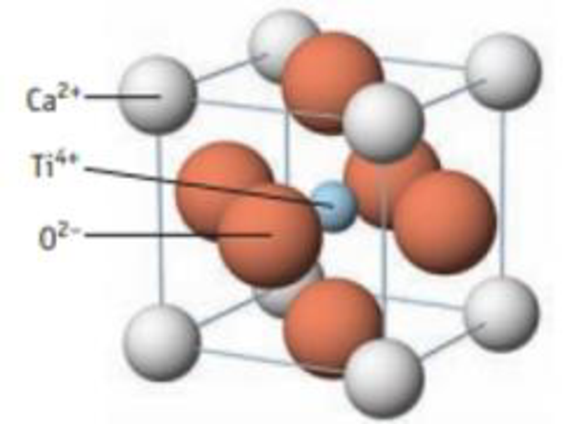Unit cell of the perovskite CaTiO3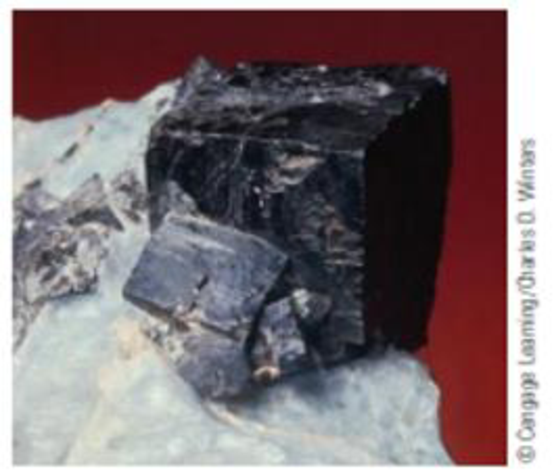A sample of perovskite, СаТiO3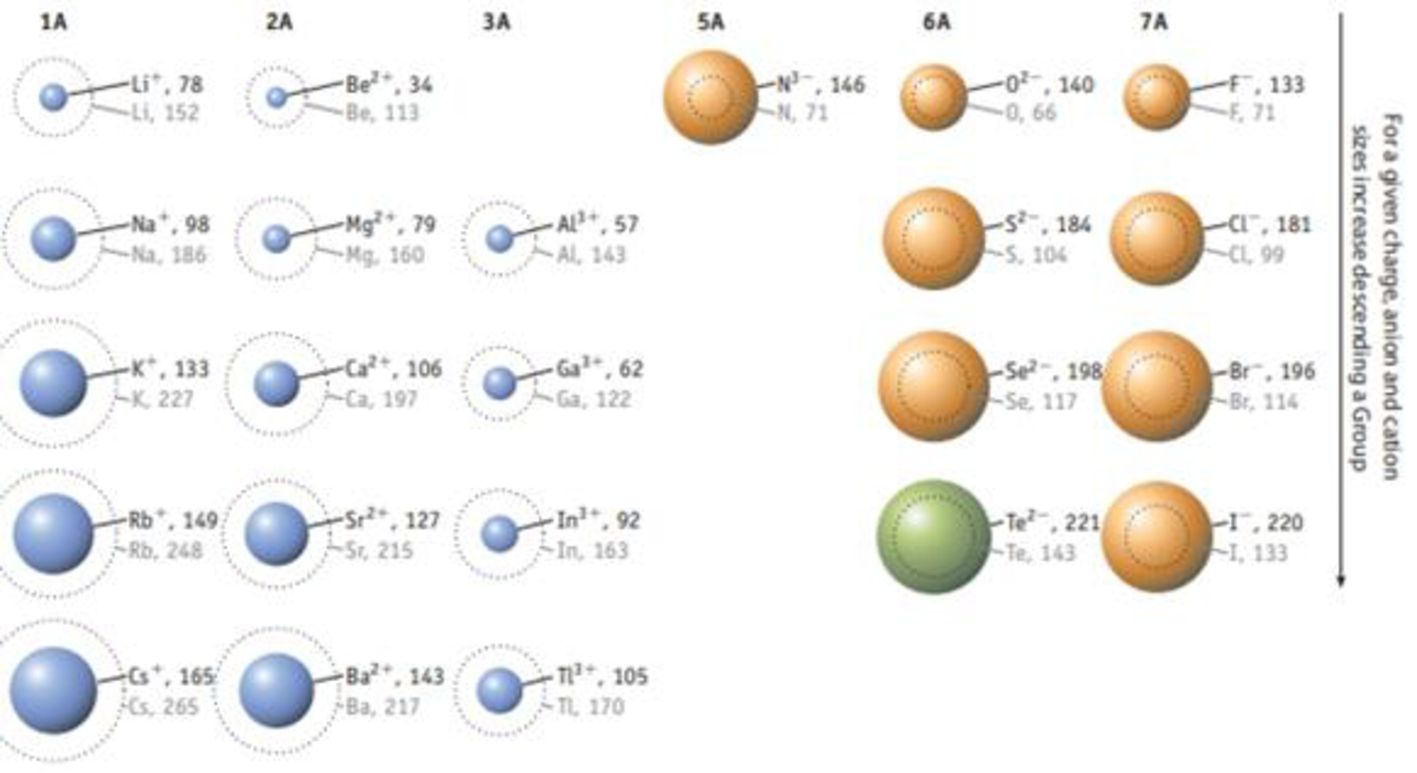FIGURE 7.11 Relative sizes of some common ions. Rodii are given in picometers (1 pm 1 × 10‒12 m). (Data taken from J. Emsley, The Elements, Clarendon Press, Oxford, 1998, 3rd edition.)

(a)

Interpretation Introduction

Interpretation:

The length of the side of the unit cell, has to be calculated.

Concept introduction:

The volume of the atom is given below,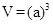The density of the unit cell is calculated as follows,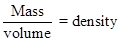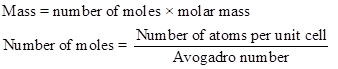Explanation

Therefore,

(b)

Interpretation Introduction

Interpretation:

The radius of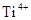in the center of the unit cell has to be calculated, and the radius ofhas to be compared with literature value.

Concept introduction:

The volume of the atom is given below,The density of the unit cell is calculated as follows,Still sussing out bartleby?

Check out a sample textbook solution.

See a sample solution

The Solution to Your Study Problems

Bartleby provides explanations to thousands of textbook problems written by our experts, many with advanced degrees!

Get Started